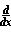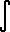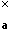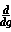Dave's Math Tables: Differentiation Identities(Math | Calculus | Derivatives | Identities)f(t) dt = f(x) (Fundamental Theorem for Derivatives)c f(x) = cf(x) (c is a constant)(f(x) + g(x)) =f(x) +g(x)f(g(x)) =f(g) *g(x) (chain rule)f(x)g(x) = f'(x)g(x) + f(x)g '(x) (product rule)

### Partial Differentiation Identities

if f( x(r,s), y(r,s) )

df / dr = df / dx * dx / dr + df / dy * dy / dr
df / ds = df / dx * dx / ds + df / dy * dy / ds

if f( x(r,s) )

df / dr = df / dx * dx / dr
df / ds = df / dx * dx / ds

directional derivative

df(x,y) / d(Xi sub a) = f1(x,y) cos(a) + f2(x,y) sin(a)
(Xi sub a) = angle counter-clockwise from pos. x axis.